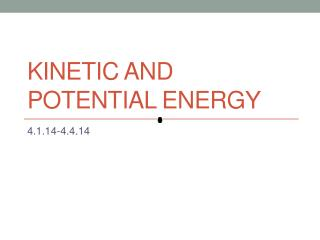DownloadDownload PresentationKinetic and Potential Energy

# Kinetic and Potential Energy

Download Presentation## Kinetic and Potential Energy

- - - - - - - - - - - - - - - - - - - - - - - - - - - E N D - - - - - - - - - - - - - - - - - - - - - - - - - - -
##### Presentation Transcript

1. Kinetic and Potential Energy 4.1.14-4.4.14

2. Kinetic Energy

3. How to think about the equation

4. Practice • A 70 kg man is walking at a speed of 2.0 m/s. What is his KE? • A 1400 Kg car is moving at a speed of 25 m/s. How much KE does the car have?

5. Potential Energy • Potential Energy- energy that is stored as a result of position or shape. • Energy is stored when a musician pulls a string. • Stored energy is converted to kinetic energy. • Two types of potential energy • Gravitational • Elastic

6. Gravitational Potential Energy • PE that depends on an objects height • A diver has a lot of PE at the top of the diving board. • GPE is dependent on mass, gravity and height • PE= mgh • The units are in Joules

7. Practice • A 60 Kg person walks from the ground to the roof of a 74.8 m tall building. How much gravitational potential energy does the person have at the top of building? • The gravitational potential energy of a diver is 3000 J. Her mass is 45 Kg. What height is she standing at?

8. Elastic Potential • Elastic Potential-is the potential energy of an object that is stretched or compressed. • We will look more at this when we get to harmonics.

9. Exit Ticket • What kind of energy is represented by an archer stretching a bow string? • What factors determine the gravitational potential of an object? • Can an object have both kinetic and potential energy? • A pitcher throws a 0.145 Kg Baseball at a velocity of 30 m/s. How much kinetic energy does the ball have?

10. Do Now 4.7.14 • Calculate the potential energy of a 45 Kg rock at the top of a 100 m hill. • A Car is driving down the road under the place where the rock is falling. It weighs 1500 Kg and is moving with a velocity of 45 m/s. What is its Kinetic Energy?

11. Energy Conservation • The law of conservation of energy states that energy cannot be created or destroyed. • All of the energy we have is present on earth it can only be converted from one form to another • This is the best evidence for ghosts!!!!

12. Potential to Kinetic • The PE of an object is converted to KE as an object falls.

13. Kinetic to Potential • Pole vaulters convert KE to PE

14. Mechanical Energy • Mechanical Energy= KE + PE • If an object has a KE of 4500J and a PE of 1000J what is the ME?

15. Conversion of ME • (KE + PE)beginning = (KE + PE)end

16. Practice • (KE + PE)beginning = (KE + PE)end • At a construction site a 1.5 kg brick is dropped from rest and hits the ground at a speed of 26 m/s. Assuming air resistance is negligible calculate the gravitational potential energy of the brick before it was dropped.

17. Practice • A 10 kg rock is dropped and hits the ground at a speed of 60 m/s. Calculate the gravitational potential energy of the rock before it was dropped.

18. Practice • A diver with a mass of 70 kg stands motionless at the top of a 3.0 m high platform. Calculate the potential energy relative to the water surface while standing on the platform, and the speed when he enters the water.

19. Practice • A pendulum with a 1 kg weight is a set in motion from a position 0.04 m above the lowest point on the path of the weight. What is the KE of the pendulum at the lowest point?

20. As you watch • Suppose you are an energy planner who is concerned about the possibility of future shortages of electricity. Write a paragraph (7 sentences) describing two proposals that you think would help to avoid this potential problem.

21. Heat • Heat is a transfer of thermal energy from one object to another. • Heat flows spontaneously from hot objects to cold objects • NOT the other way around.

22. Temperature • Temperature is a measure of how hot or cold an object is compared to a reference point. • Freezing 0 degrees • Boiling 100 degrees • Temperature is related to the average kinetic energy of the particles in an object due to their random motions through space.

23. Thermal Expansion • Occurs when particles move farther apart as temperature increases. • How does this relate to kinetic energy?

24. Specific Heat • Specific Heat- the amount of heat needed to raise the temperature of one gram of a material by one degree Celsius. • The lower a materials specific heat the more its temperature rises when a given amount of energy is absorbed by a given mass.